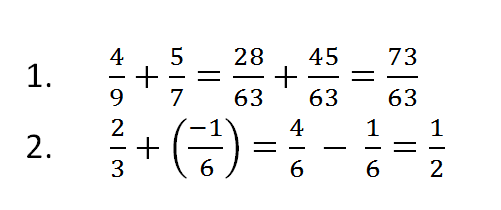Problems

# Testing

This year Andrew must pass testing at school including math course. He aggressively prepare himself - he already solves complex trigonometric equations easily do stereometry, but with rational numbers (fractions) things are bad, especially with their addition. He even afraids to make a mistake in writing the testing program. Do it for him. Recall that a rational number is defined as an irreducible fraction, where the numerator is an integer, and the denominator is a natural number.

#### Input

Four integer - the numerator and denominator of the first fraction and the numerator and denominator of the second fraction. All numbers are not greater 105 by absolute value.

#### Output

Print the numerator and denominator of the fraction's sum.\

Time limit 1 second
Memory limit 128 MiB
Input example #1
4 9 5 7


Output example #1
73 63

Input example #2
2 3 -1 6
Output example #2
1 2

Source II этап (городские и районные туры Днепропетровской области) 2014-2015 г.